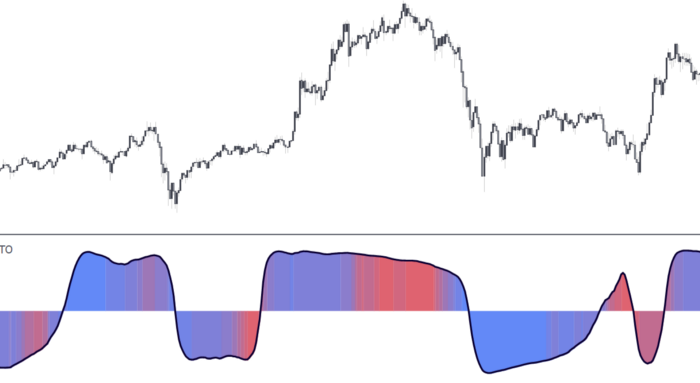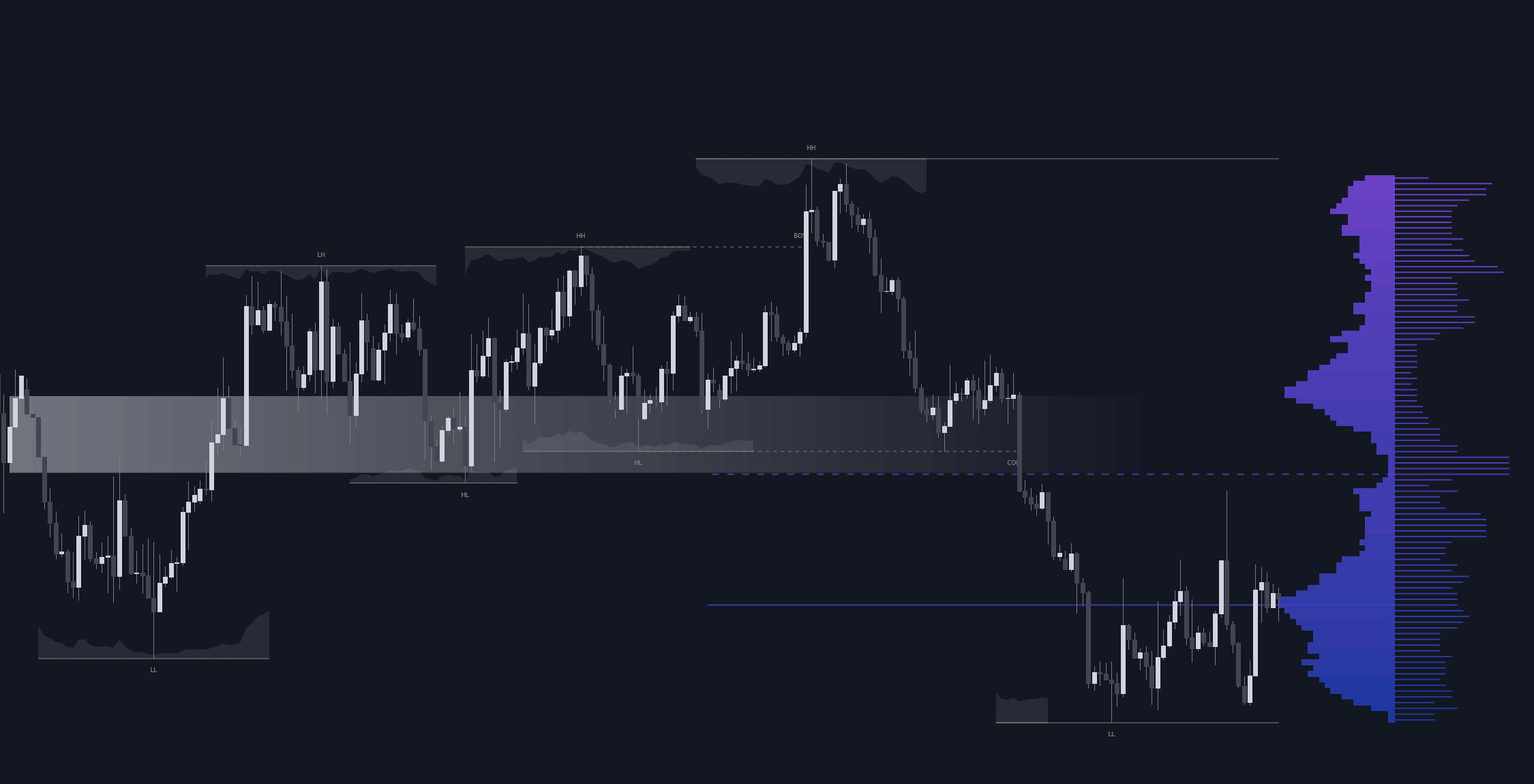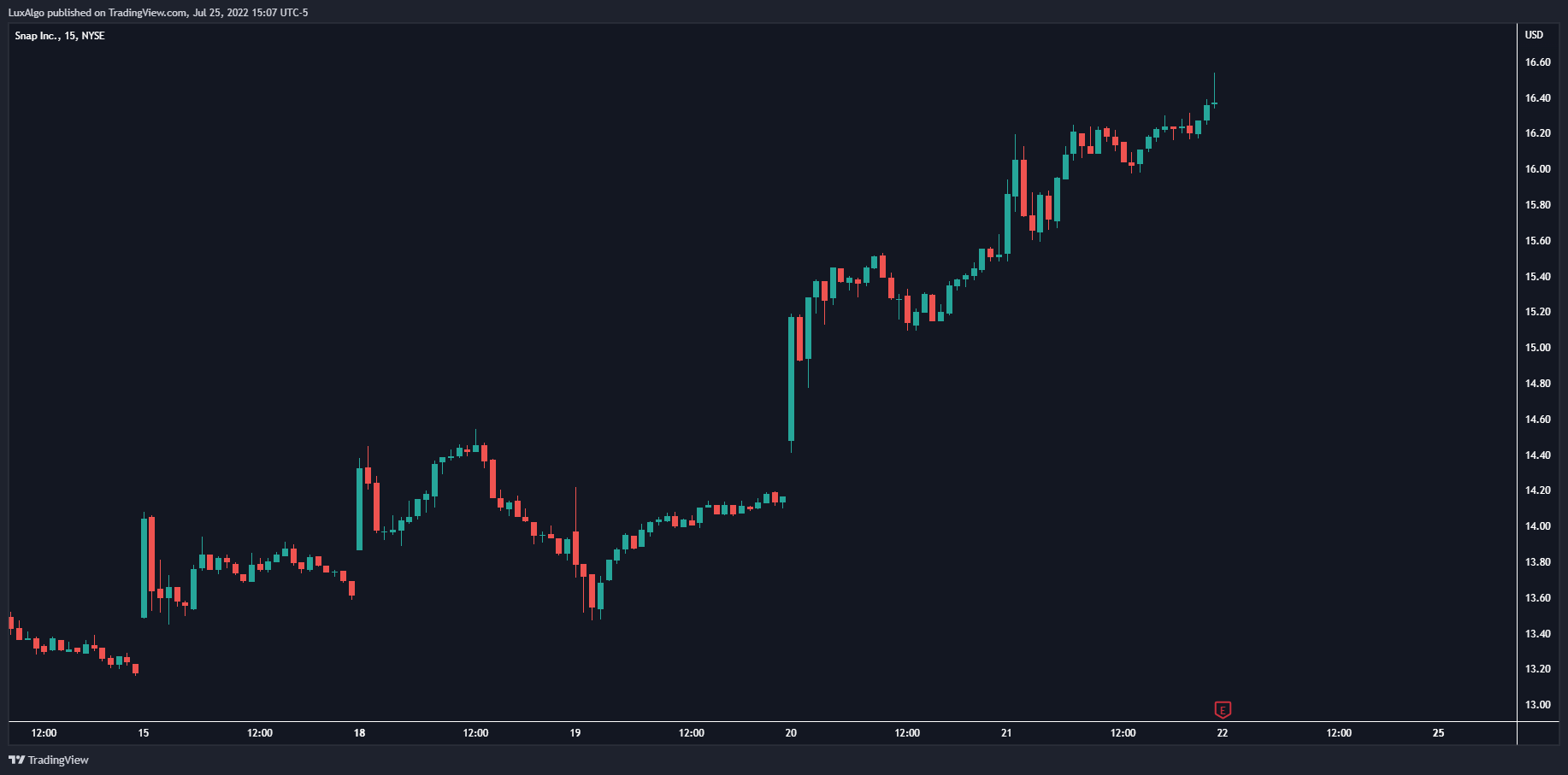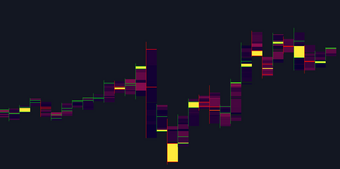# ArcTan Oscillator — A New Indicator For Technical Analysis

The following indicator is a normalized oscillator making use of the arc tangent sigmoid function (ArcTan), this allows to “squarify” the output result, thus visually filtering out certain variations originally present in the oscillator.

The following indicator is a normalized oscillator making use of the arc tangent sigmoid function (ArcTan), this allows to “squarify” the output result, thus visually filtering out certain variations originally present in the oscillator. The magnitude of this effect can be controlled by the user. The indicator contains a gradient that shows the possibility of a reversal, with red colors indicating that a reversal might occur.

### The Arctan Function

The arc tangent function (arctan) is a trigonometric function that has a characteristic “S” shaped, this is why the arctan function is a Sigmoid function. This function is also one of the many activation functions used in neural networks.

The useful characteristic of such function in technical analysis is that the arctan function will squarify any input going trough it, this effect will be more visible with inputs having a higher amplitude.

### Settings

• Length: Period of the oscillator
• Pre-Gain : Changes the amplitude of the oscillator before passing through the ArcTan function, this allows to amplify/reduce the "squarification" effect introduced by this function. In order to make it easier for the user, the setting is in a (-10,10) range, with negative values reducing the amplitude and positive one increasing it.
• Src : Source input of the indicator

### Usage

The oscillator can be used to determine the direction of the trend by looking at its sign, if the oscillator is positive, market is up-trending, else down-trending, based on this usage the user might not be interested to look at every variations produced by the oscillator, this is where the hyperbolic tangent function and pre-gain setting can be useful, by using an high value of pre-gain the user will be able to only focus on the sign of the oscillator.

Here pre-gain is set to 5, we can see that the oscillator is now easier to visualize. However, the use of sigmoid functions remove useful information for a trader that needs to find divergences, this is where using a negative value of the pre-gain setting will result useful.

The indicator makes use of a gradient to show potential reversals, this gradient is determined by the correlation between the oscillator and the price (this is a way to measure potential divergences). If the color is closer to red it means that a potential reversal might occur, it is possible to say in which direction price might go by looking at the sign of the oscillator, so if the gradient is red and the oscillator is negative price might rise. The gradient is not affected by the pre-gain setting.

## Recent posts### Smart Money Concepts (SMC) Explained for Trading Forex, Stocks, & Crypto

##### September 9, 2022### The Best Time Frame for Trading (Actual Truth)

##### August 27, 2022### Liquidity Heatmap LTF

##### May 31, 2022